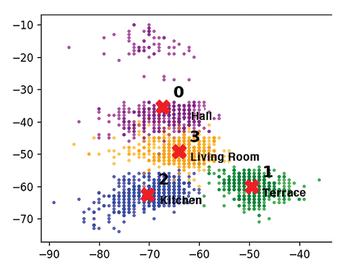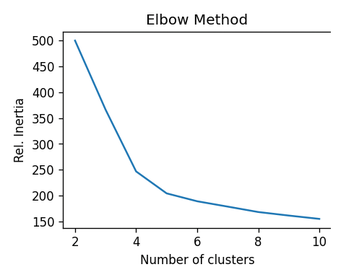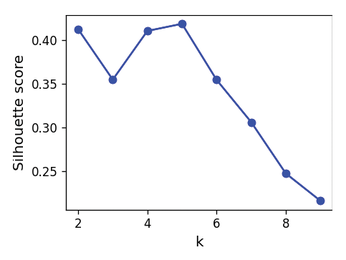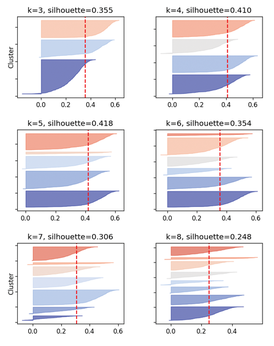## Indoor navigation with machine learning

#### Evaluating the Properties

There is a reason why many ensemble learning methods rely on decision trees. Decision trees are robust against outliers, and they process categorical data that does not need to be metrically related. You do not need to scale the data. And, incidentally, they prioritize attributes. Listing 6 finds a relative importance of 9.0 percent for the second attribute, WLAN 1, and 7.7 percent for WLAN 6. The query feature_importances_<0.1 assigns these two indices to the variable csel, which then reduces the output data by just these two columns. Repeating the calculations above with the adjusted data yields a similar result: Tom is in the living room with a probability of 89 percent.

Listing 6

Prioritizing the Properties

fi = classifier.feature_importances_
print('Feature importance: ', fi)
csel = np.where(fi<0.09)
df.drop(df.columns[csel], axis=1, inplace=True )
df
# output:
# Feature importance:
# array([0.25384511, 0.00911624, 0.09055321,
# 0.21282642, 0.24906961, 0.1073945, 0.07719491])

Listing 7

Converting the Euclidean Distance

def dbm2DistanceConverter(rssi, db0 = -20, N = 4):
'''
Input: mesured power RSSI in dBm; db0 power in 1m distance; N attenuation exponent
Output: distance in meters
formula: Distance = 10   ((db0 - RSSI)/(10 * N))
'''
# free space path loss: N=2
# reduced path loss: N>2
return 10   ((db0 - rssi )/(10 * N))
def eucV(p,b):
"""Euclidean distance between two points squared"""
return (p-b) 2 + (p-b) 2

Redundant data does not affect the accuracy of machine learning training because detecting redundancy is part of the training. This is different if redundant data slows down the learning process or feeds in attributes with contradictory data. Later, I will cover other methods that do not simply delete attributes but try to combine them.

#### Unsupervised Learning

Until now, I have assumed that I know the location for each measurement. But what if I was careless when noting down the rooms? Unsupervised learning looks for statements that can be derived from the data without contradiction. In this case, unsupervised learning would group similar measurements together and assume a common origin. However, whether Tom's location is the kitchen or the living room remains undetermined.

Like in Listings 1 and 3 using supervised learning, the data ends up in an array (Listing 8). To distinguish the data, I use Xu instead of X. The square brackets [:,:-1] delete the target size in the last column. To compare the data, I later resort to the pandas DataFrame df from supervised learning.

Listing 8

Output Data Without a Location

import numpy as np
import pandas as pd
import matplotlib.pyplot as plt
fn = "images/wifi_localization.txt"
#fn = "https://archive.ics.uci.edu/ml/machine-learning-databases/00422/wifi_localization.txt"
Xu = np.loadtxt(fn)[:,:-1]

In my experiments here, I am restricting myself to the K-Means classifier . The letter K expresses the similarity to the k-nearest neighbor algorithm, which searches for the k nearest neighbors, where k stands for the number. K-Means divides the data into k classes and optimizes the number of k centroids such that the sum of the squared distances of the points to their respective centroids remains minimal. Although it sounds a bit abstract, this can be programmed with just a few lines of code thanks to scikit-learn  (Listing 9).

Listing 9

The K-Means Classifier

01 from sklearn.cluster import KMeans
02 clusters = 4
03 kmeans = KMeans(n_clusters=clusters, init='k-means++', max_iter=300, n_init=10, random_state=0)
04 kmeans.fit(Xu)
05 y_pred = kmeans.predict(Xu)
06 clusterCenters = kmeans.cluster_centers_

Line 1 in Listing 9 imports the classifier and line 3 sets up the hyperparameters. The classifier needs to know the number of clusters; I will choose 4 for now. The other parameters are default values. k-means++ helps the software find good initial values, which it optimizes in max_iter steps. It makes n_init attempts and selects the best solution. random_state starts the pseudorandom generator at a defined point, which means that each iteration of the computations returns an identical result.

Line 5 shows the fruits of my labor: The trained method uses kmeans.predict(Xu) to assign the measurements to the clusters (i.e., in the case of four clusters, one of the numbers 0, 1, 2, or 3). The seven coordinates of the four clusters' focal points are stored in the method variable cluster_centers_.

Listing 10 visualizes the result (Figure 10). Strictly speaking, Figure 10 is just a projection of the seven-dimensional property space onto a two-dimensional drawing plane. The choice of the   and 4 columns in line 1 is not entirely accidental: They contain the high-priority features found during supervised learning. When I look at principal component analysis (PCA)  later, I will discover another – also unsupervised – selection method.

Listing 10

Visualizing the K-Means Result

01 x1, x2 = 4, 0
02 colormap = np.array(['purple', 'green', 'blue', 'orange'])
03 plt.figure(figsize=(6,4), dpi=120)
04 plt.scatter(Xu[:,x1], Xu[:,x2], s= 10, c=colormap[y_pred])
05 plt.scatter(clusterCenters[:, x1], clusterCenters[:, x2], s=180, c='red', marker = 'X')
06 for i, p in enumerate(clusterCenters):
07   plt.annotate(f'$\\bf{i}$', (p[x1]+1, p[x2]+3))
08 plt.show()Figure 10: Visualizing the four clusters found by K-Means.

The plt.scatter instruction prints all the measured values, selecting the colors from the colormap (line 4). The index for the color is the y_pred set in Listing 9. The focal points are marked as red crosses by the second scatter command in line 5.

Unsupervised learning divides the data into groups and chooses the assignments randomly. In Listing 9, if the initial value of the random random_state were not fixed, the groups would get different numbers each time they ran – I'll come back to that later.

#### Hidden Spaces

Listing 11 provides statistical information about the assignment's quality; Listing 12 shows a typical output. The output's inertia says something about a cluster's compactness. The points should be grouped as tightly as possible around the cluster's focal point: The smaller the value for the same number of points, the better. The output's silhouette takes into account the distance to the neighboring clusters. The farther away the neighboring clusters are, the clearer the delineation of a cluster. The silhouette value lies between 1 (optimal) and -1 (possibly wrongly set cluster focal points). Both values describe the tendency in comparison with different cluster sizes with identical initial data.

Listing 11

K-Means Results

from sklearn.metrics import silhouette_score
print(f'Input data shape: {Xu.shape}')
print(f'Inertia:  {kmeans.inertia_:3.1f}')
print(f'Silhouette: {silhouette_score(X, kmeans.labels_)}')
print(f'New labels: {np.unique(kmeans.labels_)}')
clusterCenters = kmeans.cluster_centers_
print(f'Center of gravity: {clusterCenters}' )

Listing 12

K-Means Typical Output

Input data shape: (2000, 7)
Inertia: 246771.6
Silhouette: 0.41023382751730914
New labels: [0 1 2 3]
Center of gravity: [[-35.43058824 ...]

Listing 13 calculates inertias for different clusters and Figure 11 plots the values. The optimum result is a small inertia for the smallest possible cluster size. The elbow method looks for the point at which the inertia's steep slope changes to a shallow slope. With a little good luck, this point will be at a cluster number of 4 or 5. Listing 14 does a similar job for calculating the silhouette; Figure 12 shows the results. Again, the best values are at 4 and 5.

Listing 13

Finding the Optimum Cluster Size

wcss = []
for i in range(2, 11):
kmeans = KMeans(n_clusters=i, init='k-means++', max_iter=300, n_init=10, random_state=0)
kmeans.fit(Xu)
wcss.append(kmeans.inertia_)
plt.plot(range(2, 11), wcss)
plt.title('Elbow Method')
plt.xlabel('Number of clusters')
plt.ylabel('WCSS')
plt.show()

Listing 14

Plotting the Mean Silhouette Value

from sklearn.metrics import silhouette_score
kmeansk = [KMeans(n_clusters=k, random_state=2).fit(Xu) for k in range(1, 10)]
inertias = [model.inertia_ for model in kmeansk]
silhouette_scores = [silhouette_score(Xu, model.labels_)  for model in kmeansk[1:]]
plt.figure(figsize=(4,3), dpi=120)
plt.plot(range(2, 10), silhouette_scores, "bo-")
plt.xlabel("Number of clusters")
plt.ylabel("Silhouette score", fontsize=12)
plt.show()Figure 11: The elbow method finds the optimum cluster size.Figure 12: The silhouette method for finding the optimum cluster size.

In Figure 13, each cluster group from k=3 to k=8 is given its own subplot. The mean values are indicated by a vertical red line. In addition, the silhouette value of each dataset appears as a horizontal bar, sorted by size. The more pointed the right end of the bar looks, the greater the variation of the values and the more nonuniform the cluster.Figure 13: The silhouette values of the individual datasets, sorted by size.

With five clusters, the maximum values are at a uniform level of almost 0.6. The second narrow bar suggests that the data contains one small cluster in addition to the four large ones. After expanding the number of clusters to five in Listing 9 (i.e., from clusters = 4), K-Means identifies the set of points at the top of Figure 10 as a separate group (i.e., a fifth room).

Unsupervised learning finds connections that would have been hidden in supervised learning. Using this method puts forth the suggestion that the data was recorded in five different rooms, not four.

Express-Checkout as PDF
Price \$2.95
(incl. VAT)

SINGLE ISSUES

SUBSCRIPTIONS

## Related content

• Perl – k-means Clusters

A human observer can register clusters in a two-dimensional set of points at a glance. Artificial intelligence has a harder time getting it done; however, the relatively simple k-means method delivers usable results.

• KNIME

They say data is "the new oil," but all that data you collect is only valuable if it leads to new insights. An open source analysis tool called KNIME lets you analyze data through graphical workflows – without the need for programming or complex spreadsheet manipulation.

• Unsupervised Learning

The most tedious part of supervised machine learning is providing sufficient supervision. However, if the samples come from a restricted sample space, unsupervised learning might be fine for the task.

• Treasure Hunt

A geolocation guessing game based on the popular Wordle evaluates a player's guesses based on the distance from and direction to the target location. Mike Schilli turns this concept into a desktop game in Go using the photos from his private collection.

• Machine Learning

"I won't make this mistake again," you promise yourself. In other words, you'll learn from experience. If you translate experience into data, computers can do that, too. We'll introduce you to the fundamental forms of machine learning.

# Support Our Work

Linux Magazine content is made possible with support from readers like you. Please consider contributing when you've found an article to be beneficial.

## News

• ### The GNU Project Celebrates Its 40th Birthday

September 27 marks the 40th anniversary of the GNU Project, and it was celebrated with a hacker meeting in Biel/Bienne, Switzerland.

• ### Linux Kernel Reducing Long-Term Support

LTS support for the Linux kernel is about to undergo some serious changes that will have a considerable impact on the future.

• ### Fedora 39 Beta Now Available for Testing

For fans and users of Fedora Linux, the first beta of release 39 is now available, which is a minor upgrade but does include GNOME 45.

• ### Fedora Linux 40 to Drop X11 for KDE Plasma

When Fedora 40 arrives in 2024, there will be a few big changes coming, especially for the KDE Plasma option.

• ### Real-Time Ubuntu Available in AWS Marketplace

Anyone looking for a Linux distribution for real-time processing could do a whole lot worse than Real-Time Ubuntu.

• ### KSMBD Finally Reaches a Stable State

For those who've been looking forward to the first release of KSMBD, after two years it's no longer considered experimental.

• ### Nitrux 3.0.0 Has Been Released

The latest version of Nitrux brings plenty of innovation and fresh apps to the table.

• ### Linux From Scratch 12.0 Now Available

If you're looking to roll your own Linux distribution, the latest version of Linux From Scratch is now available with plenty of updates.

• ### Linux Kernel 6.5 Has Been Released

The newest Linux kernel, version 6.5, now includes initial support for two very exciting features.

• ### UbuntuDDE 23.04 Now Available

A new version of the UbuntuDDE remix has finally arrived with all the updates from the Deepin desktop and everything that comes with the Ubuntu 23.04 base.